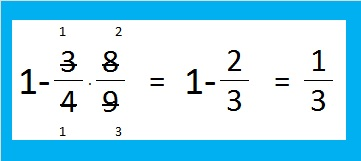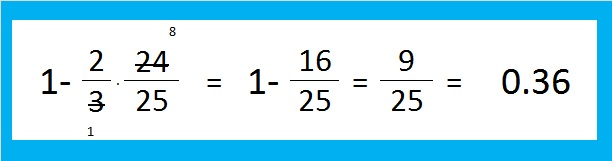# 252 How likely can this square root be simplified?

• 252 is a composite number.
• Prime factorization: 252 = 2 x 2 x 3 x 3 x 7, which can be written 252 = (2^2) x (3^2) x 7
• The exponents in the prime factorization are 2, 2, and 1. Adding one to each and multiplying we get (2 + 1)(2 + 1)(1 + 1) = 3 x 3 x 2 = 18. Therefore 252 has 18 factors.
• Factors of 252: 1, 2, 3, 4, 6, 7, 9, 12, 14, 18, 21, 28, 36, 42, 63, 84, 126, 252
• Factor pairs: 252 = 1 x 252, 2 x 126, 3 x 84, 4 x 63, 6 x 42, 7 x 36, 9 x 28, 12 x 21, or 14 x 18
• Taking the factor pair with the largest square number factor, we get √252 = (√7)(√36) = 6√7 ≈ 15.875The square root of a whole number can be simplified if it has a square number factor. How likely is that condition met by any random whole number?

4 is 2 x 2 and therefore a square number.  1 out of every four whole numbers (or 25%) is divisible by 4

3^2 = 9. Likewise 1 out of every nine whole numbers is divisible by square number 9 (about 11.1%).

Some numbers, like 252, are divisible by both 4 and 9. (1 out of every 36 numbers are divisible by both 4 and 9.)Thus 1/3 of all whole numbers are divisible by 4, 9 or both.

That means that 2/3 of the numbers in the set of all whole numbers are NOT divisible by 4, 9 or both. It is often easier to compute the probability of something NOT happening and then subtract that fraction from 1 to determine the probability of something actually happening. The probability a number is NOT divisible by 4 is 3/4 while the probability a whole number is NOT divisible by 9 is 8/9. We get the same result either way.1/3 of all whole numbers (about 33.3%) are divisible by either 4 or 9! That fact is very cool because it is so easy to tell if a number is divisible by 4 or 9: If the last 2 digits of a number is divisible by 4, the entire number is divisible by 4 and if the sum of the digits of a whole number is divisible by 9, that whole number is divisible by 9.

It is also very easy to tell if a number is divisible by 5 x 5 or 25. If the last two digits of the number are 25, 50, 75 or 00, then it is divisible by 25. Let’s compute how likely it is that the square root of a number can be simplified because that number is divisible by 4, 9, or 25.Thus 36% of all whole numbers are divisible by 4, 9, or 25 and therefore have square roots that can be simplified! It is not as easy to tell if a number is divisible by 49, 121, 169, or any other number that is the perfect square of a prime number. The percentage of numbers that are divisible by these other perfect squares doesn’t go up much more either. Consider this infinite product subtracted from 1:When I’ve computed the partial product up to 3480/(59 x 59) and subtracted it from 1, the probability only increased to 39.010%. I used excel to compute the probability of a number being divisible by a square factor up to 1,495,729 (which is 1223^2) and it is only 39.201%. There isn’t much change in the percentage between the 17th prime number (59) and the 200th prime number (1223).

As n gets larger (n^2 -1)/(n^2) gets closer and closer to 1. I conclude that the probability that a random whole number can have its square root simplified is about 40%.